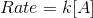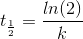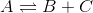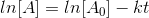# AP Chemistry : Principles of Reaction Kinetics

## Example Questions

### Example Question #1 : Principles Of Reaction Kinetics

The overall reaction can only proceed as quickly as the __________.

products are removed

rate-determining step

intermediate is decomposed

rate-determining step

Explanation:

The rate-determining step in a reaction mechanism is a kinetic bottleneck, in that it prevents the overall reaction from proceeding; thus, it is what determines how quickly the overall reaction can proceed.

### Example Question #2 : Principles Of Reaction Kinetics

Which of the following does not affect a forward reaction rate?

Product concentration

Temperature

Catalysts

Medium

Product concentration

Explanation:

Product concentration would not affect a forward reaction rate, since that is what is being formed. Catalysts specifically speed up reaction rates, as does temperature. Medium can also affect reaction rate because some molecules are more likely to react with each other in certain environments.

### Example Question #3 : Principles Of Reaction Kinetics

Which of the following is not true of the transition state?

It has a greater energy than the products

It has a greater energy than the reactants

It cannot dissociate into products without needing any additional energy

Old bonds are weakened and new bonds begin to form

It cannot dissociate into products without needing any additional energy

Explanation:

The transition state is the energy barrier in a reaction—energy is needed to reach this state. Once it is acheived, however, it can either revert back to reactants or dissociate into products without any added energy.

### Example Question #4 : Principles Of Reaction Kinetics

Which of the following is true:

I. First order reactions proceed at a constant rate

II. First order reactions have a constant half-life

III. First order reactions have one reactant

II and III

I only

I, II, and III

II only

II only

Explanation:

First order reactions have the rate equation. As the molarity of A decreases, the rate slows.

The half-life equation is. The half-life is constant with respect to time and concentration, depending only on the rate constant.

Lastly, the overall reaction can have any number of reactants. A reaction is first order because its rate-limiting step has only one reactant.

Only statement II is true.

### Example Question #5 : Principles Of Reaction Kinetics

The following reversible reaction takes place in a closed container:The system is allowed to reach equilibrium before 2 moles of reactant A are added to the container.

The net rate of formation of product B immediately following the addition of reactant A is __________.

impossible to determine without additional information

zero

negative

positive

positive

Explanation:

According to Le Chatelier's principle, an increase in the concentration of reactants will cause the reaction to shift to the right in order to re-establish equilibrium. Thus, immediately after reactant A is added, the forward reaction will increase and the net rate of formation of the products will be positive.

### Example Question #6 : Principles Of Reaction Kinetics

Rate data is collected for a reaction, and the following integrated rate law is derived.If this reaction is __________ with respect to reactant A, a plot of ln[A] versus time would be __________.

second order . . . parabolic

first order . . . parabolic

zero order . . . linear

first order . . . linear

first order . . . linear

Explanation:

The integrated rate law shown is for a reaction rate law with a first-order dependence on reactact A. If such a rate law accurately describes the kinetics of the reaction, then ln[A] will vary linearly with respect to time.

### Example Question #7 : Principles Of Reaction Kinetics

Which of the following affects the rate of a reaction?

Frequency of collision

Molecules' energies

All of these

Collision orientation